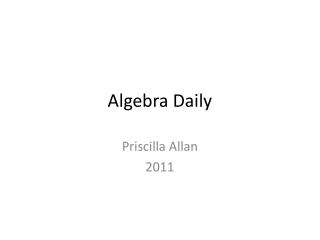DownloadDownload PresentationAlgebra Daily

# Algebra Daily

Télécharger la présentation## Algebra Daily

- - - - - - - - - - - - - - - - - - - - - - - - - - - E N D - - - - - - - - - - - - - - - - - - - - - - - - - - -
##### Presentation Transcript

1. Algebra Daily Priscilla Allan 2011

2. Do as many as you can: Given 3 + a = -1 find the value of a Given 9 + b = -10 find the value of b Given 30 -c = 13 find the value of c Given 9d = -18 find the value of d Given 3/e = -1 find the value of e Given f/2= -11 find the value of f Given 6g + 4= -8 find the value of g

3. Solve by forming equations I go to the shop to buy pens and pencils. I buy 3 more pens than pencils. The pencils cost \$1 and the pens cost \$2 each. The total price paid is \$27. How many pens do I buy? Let x represent the number of pencils Let y represent the number of pens x+3=y and 1x+2y=27 x+2(x+3)=27; x+2x+6=27; 3x=21; x=7; y=10 You purchased 10 pens and 7 pencils.

4. DO THIS NOW! I go to the shop to buy pens and pencils. I buy 5 more pens than pencils. The pencils cost \$1.50 and the pens cost \$2.30 each. The total price paid is \$45.70. How many pens do I buy? 9 pencils and 14 pens Extra for those who have finished Solve 3x2 – 8 = 10x

5. Extra for those who have finishedSolve 3x2 – 8 = 10x 3x2 – 8 = 10x (rearrange to see quadratic form) 3x2- 10x – 8 = 0 Multiply the 3 and the -8 to get -24 Look for two numbers that add to -10 and multiply to -24. -12+2=-10 and -12*2=-24 THEN Replace -10x with -12x + 2x. 3x2- 12x + 2x – 8 = 0 (now factor in pairs) 3x(x – 4) + 2(x – 4) = 0 (3x + 2)(x – 4) = 0 Either 3x + 2=0 OR x – 4 = 0 So x = -2/3 or x = 4

6. The order does not matter when replacing the x term. 3x2- 10x – 8 = 0 Multiply the 3 and the -8 to get -24 Look for two numbers that add to -10 and multiply to -24. The numbers are -12 and 2 -12+2=-10 and -12*2=-24 THEN Replace -10x with 2x – 12x 3x2+ 2x - 12x – 8 = 0 (now factor in pairs) x(3x+2) – 4(3x+2)=0 (x-4)(3x+2)=0 Either 3x + 2=0 ORx – 4 = 0 Sox = -2/3 or x = 4